# Revisal Problems (Past 13 Years) JEE Main (Thermodynamics)

## 20 Questions MCQ Test Chemistry for JEE Advanced | Revisal Problems (Past 13 Years) JEE Main (Thermodynamics)

Description
Attempt Revisal Problems (Past 13 Years) JEE Main (Thermodynamics) | 20 questions in 40 minutes | Mock test for JEE preparation | Free important questions MCQ to study Chemistry for JEE Advanced for JEE Exam | Download free PDF with solutions
QUESTION: 1

Solution:
QUESTION: 2

Solution:
QUESTION: 3

### In the following reaction,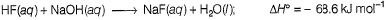This value is much higher than the heat of neutralisation of strong acid with strong base (-57.3 kJ mol-1) . This is because

Solution:
QUESTION: 4

For the above reaction, 2NO(g) + O2(g) → 2NO2(g)

Select incorrect relation

Solution:
QUESTION: 5

At what temperature will the equilibrium constant for the formation of NOCI(g) be

Keq = Kp = 1.0 x 103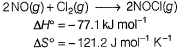Solution:
QUESTION: 6

For the reaction at 298 K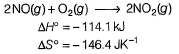Thus ΔG° for the given reaction is

Solution:
QUESTION: 7

Methane is a commercial source of H2 through the reaction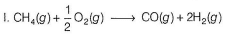Based on the following thermochemical equations (II to IV)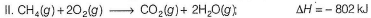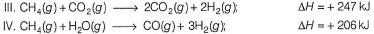Q. ΔH of equation (I) is

Solution:
QUESTION: 8

Given,

Thus, for the reaction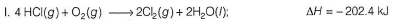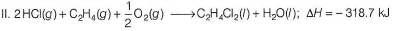Thus, for the reaction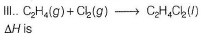Solution:
QUESTION: 9

Vapour pressure of a liquid is 10 mm at 300 K, 20 mm at 400 K. What is the vapour pressure at 500 K?

Solution:
QUESTION: 10

The combustion of hydrogen-oxygen mixture is used to produce very high temperatures (= 2500 °C) needed for certain types of welding operations.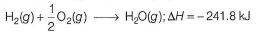Q. Quantity of heat, (in kJ) evolved when a 180 g mixture containing equal parts of H2 and O2 mass is burned, is

Solution:
QUESTION: 11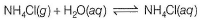Due to dissolution of ammonium chloride in H2O, temperature falls rapidly. Thus,

Solution:
QUESTION: 12

The standard heat of combustion of benzoic acid, C6H5COOH(s), at 1 bar and 298 K is -329.3 kJ mol-1. The standard heat of combustion at constant volume is

Solution:
QUESTION: 13

Bond dissociation energies of H—H, O=O, O—H and O—O are x1, x2, x3, and x4 respectively.

Thus, heat of formation of H2O2(g) is

Solution:
QUESTION: 14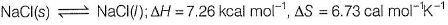Thus, melting point of NaCI (s) is

Solution:
QUESTION: 15

Quantity of work (in joules) done by the gas if it expands against a constant pressure of 0.980 atm and the change in volume (Δ/) is 25.0 L, is

Solution:
QUESTION: 16

The combustion of 1.0 g of sucrose (C12H22O11) in a bomb calorimeter causes the temperature to rise from 25.0°C to 28.5°C. The heat of combustion of sucrose is (heat capacity of the calorimeter system is 4.90 kJ K-1

Solution:
QUESTION: 17

A gas, while expanding absorbs 25 J of heat and does 243 J of work. Thus, ΔE (change in internal energy) for the gas is

Solution:
QUESTION: 18

1.0 L of liquid water freezes under a constant pressure of 1.0 atm and forms 1.1 L of ice.

Thus, work done is

Solution:
QUESTION: 19

In the following adiabatic expansion of 1 mole of CO2 gas, temperature T is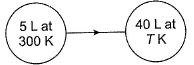Solution:
QUESTION: 20

For the dissociation of PCI5(g)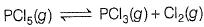Slope of the linear curve is such that θ = tan-1 (-1.5)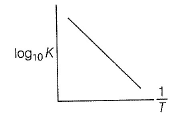Q. Thus, ΔH° is

Solution: Tan (theta) =-1.5, according to the graph, tan (theta) =logk/1/T=Tlogk , and we know, dH=-2.303×dn×RTlogk=-2.303×1×8.31×(-1.5)=28.72Use Code STAYHOME200 and get INR 200 additional OFF Use Coupon Code